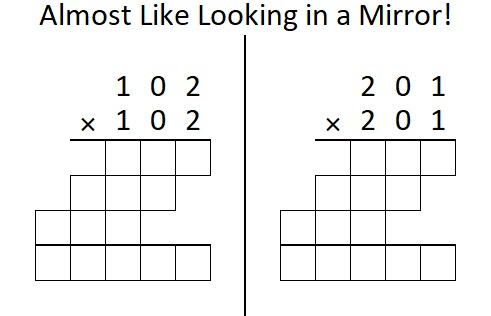# Math Happens When Two of 1632’s Factors Look in a Mirror!

Contents

### Today’s Puzzle:

Both 12 and 102 are factors of 1632. Something special happens when either one squares itself and looks in a mirror. Solving this puzzle from Math Happens will show you what happens to 12 and 12².

You can see that puzzle on page 33 of this e-edition or this pdf of the Austin Chronicle. You can find other Math Happens Puzzles here.

This next puzzle will help you discover what happens when 102 and 102² look in a mirror!Why do you suppose the squares of (12, 21) and (102, 201) have that mirror-like property?

### Factor Trees for 1632:

There are many possible factor trees for 1632, but today I will focus on two trees that use factor pairs containing either 12 or 102:### Factors of 1632:

• 1632 is a composite number.
• Prime factorization: 1632 = 2 × 2 × 2 × 2 × 2 × 3 × 17, which can be written 1632 = 2⁵ × 3 × 17.
• 1632 has at least one exponent greater than 1 in its prime factorization so √1632 can be simplified. Taking the factor pair from the factor pair table below with the largest square number factor, we get √1632 = (√16)(√102) = 4√102.
• The exponents in the prime factorization are 5, 1, and 1. Adding one to each exponent and multiplying we get (5 + 1)(1 + 1)(1 + 1) = 6 × 2 × 2 = 24. Therefore 1632 has exactly 24 factors.
• The factors of 1632 are outlined with their factor pair partners in the graphic below.### More about the Number 1632:

1632 is the hypotenuse of a Pythagorean triple:
768-1440-1632, which is (8-15-17) times 96.

1632 is the difference of two squares in EIGHT different ways:
409² – 407² = 1632,
206² – 202² = 1632,
139² – 133² = 1632,
106² – 98² = 1632,
74² – 62² = 1632,
59² – 43² = 1632,
46² – 22² = 1632, and
41² – 7² = 1632.

That last difference of two squares means 1632 is only 49 numbers away from the next perfect square, 1681.

This site uses Akismet to reduce spam. Learn how your comment data is processed.